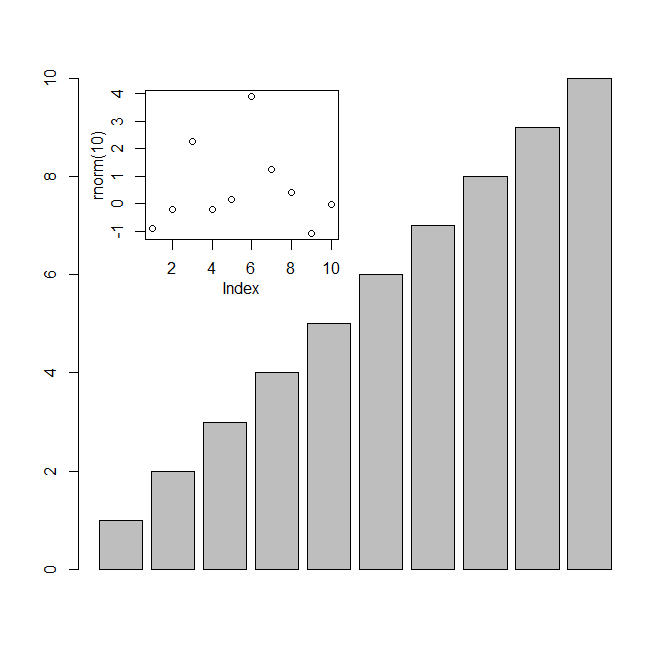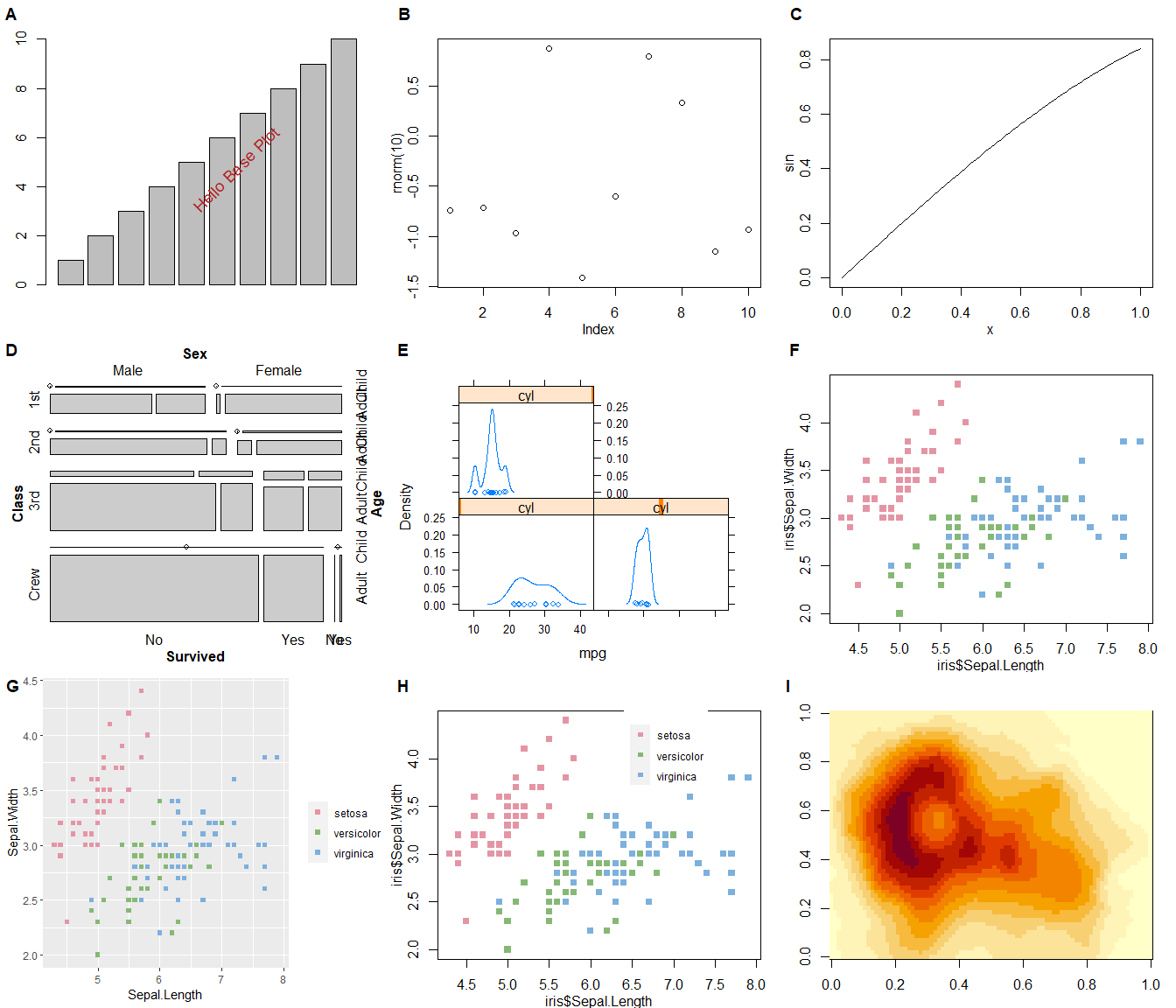## Warning: package 'cowplot' was built under R version 4.0.3

## grid

library("grid")
library("ggplotify")

p1 <- as.grob(~barplot(1:10))
p2 <- as.grob(expression(plot(rnorm(10))))
p3 <- as.grob(function() plot(sin))

library("vcd")
data(Titanic)
p4 <- as.grob(~mosaic(Titanic))

library("lattice")
data(mtcars)
p5 <- as.grob(densityplot(~mpg|cyl, data=mtcars))

as.grob function accepts plot function call as expression or formula, or a function that plots to an R graphics device. The plots can be generated by base graphics (p1, p2, p3) or grid (p4). If the plot function produce graphic object, it can be directly used as input (p5, can be trellis object by lattice package, meme object by meme package, upset object by ‘UpSetR’ package, etc.).

It will convert the plot to grob object, so that it can be compatible with grid system and related packages.

We can now use grid.draw to plot p1 and p2, and use pushViewport to embed plot inside another plot.

grid.newpage()
grid.draw(p1)
vp = viewport(x=.35, y=.75, width=.35, height=.3)
pushViewport(vp)
grid.draw(p2)
upViewport()If you are not family with grid, you can use ggplot2 to do similar task (e.g. p8 in the below example).

## ggplot2

All the plots that can be converted to grob are supported to be converted to ggplot object by using as.ggplot function.

library(ggplot2)
p1 <- as.ggplot(~barplot(1:10)) +
annotate("text", x = .6, y = .5,
label = "Hello Base Plot", size = 5,
color = 'firebrick', angle=45)

p2 <- as.ggplot(expression(plot(rnorm(10))))
p3 <- as.ggplot(function() plot(sin))

p4 <- as.ggplot(~mosaic(Titanic))

p5 <- as.ggplot(densityplot(~mpg|cyl, data=mtcars))

## Align plots

With all the plots converted to ggplot objects, we are able to align plots produced by ‘base’ and ‘grid’ graphic systems using cowplot or patchwork.

library(cowplot)

library(colorspace)
col <- rainbow_hcl(3)
names(col) <- unique(iris$Species) color <- col[iris$Species]
p6 <- as.ggplot(~plot(iris$Sepal.Length, iris$Sepal.Width, col=color, pch=15))

p7 <- ggplot(iris, aes(Sepal.Length, Sepal.Width, color=Species)) +
geom_point(shape=15) + scale_color_manual(values=col, name="")

legend <- get_legend(p7)

## also able to annotate base or other plots using ggplot2
library(ggimage)
p8 <- p6 + geom_subview(x=.7, y=.78, subview=legend)

p9 <- as.ggplot(~image(volcano))

plot_grid(p1, p2, p3, p4, p5, p6, p7, p8, p9, ncol=3, labels=LETTERS[1:9])